# Lateral Flow Assay Model

We proposed to use a lateral flow device to detect the labelled RNA, as stated in design page. We can reasonably assume that liquids will do one-dimensional diffusion in our lateral flow device since the width (y) and the height (z) of the device can be ignored compared to its length, as Fig.1 shows: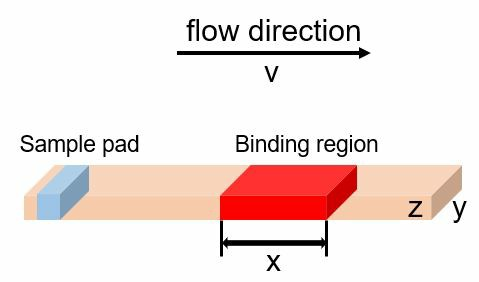Fig.1 The schematic diagram for lateral flow assay device

Now let’s consider the antibody-protein binding kinetics. There are two processes at work: the formation of protein-antibody complex (PA) from protein (P) and antibody (A), and the dissociation of the complex into protein and antibody. We can therefore use equation 2.1 to depict the binding kinetics:where r is the net rate for protein-antibody complex formation, ka, kb are the reaction kinetic constant for complex formation and dissociation. Cp,A,pA is the concentration of corresponding protein. When the reaction is at equilibrium, note that the reaction rate r can be depicts in another way:Now let’s consider the protein concentration inside the binding area. Assume the length of the binding region is x, then we will have the volume of the binding region (V):Assume the flow rate of the liquid is v, then we have the volume of the flow into the region per unit time (D):According to law of conservation of mass, the difference of the protein amount between inflow and outflow equals to the absorbed protein amount by the antibody:Combine equation 2.1~2.4 with 2.5, we have:where CA,0 is the antibody amount before protein-antibody binding.

We can derive the expression of protein concentration in outflow, Cp,out, from equation 2.6:Let v/x = t, we can calculate the derivate of Cp,out versus t: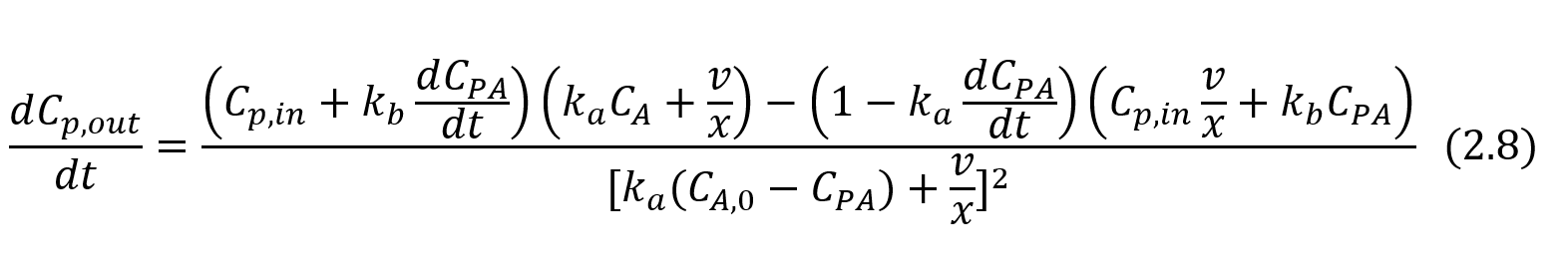Apparently the denominator or the right side of equation 2.8 is no less than 0. So we can discuss the numerator alone to decide the sign of the derivate of Cp,out versus t

Rewrite the numerator part: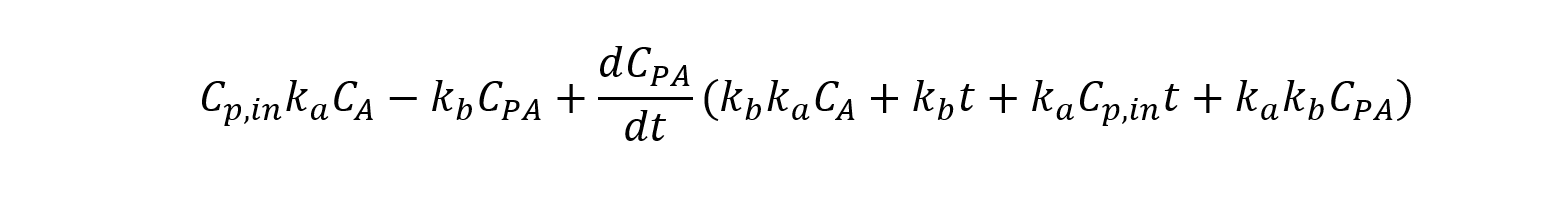Note that r = kaCpCA - kbCPA ≥ 0, and Cp,in ≥ Cp, therefore: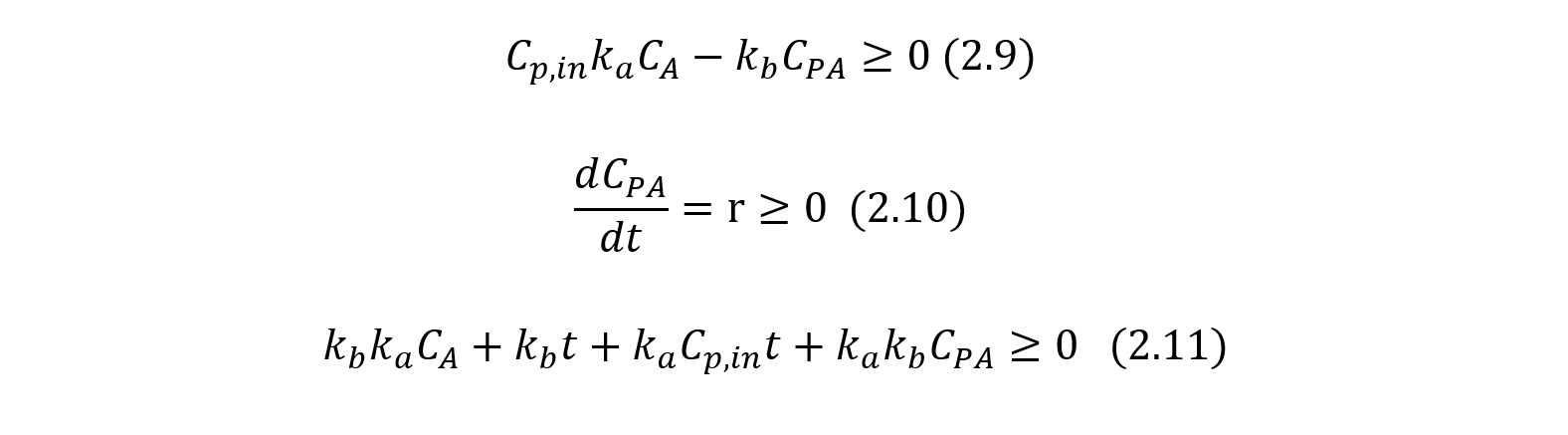therefore we have:Combiner inequation 2.9 and 2.12, we have: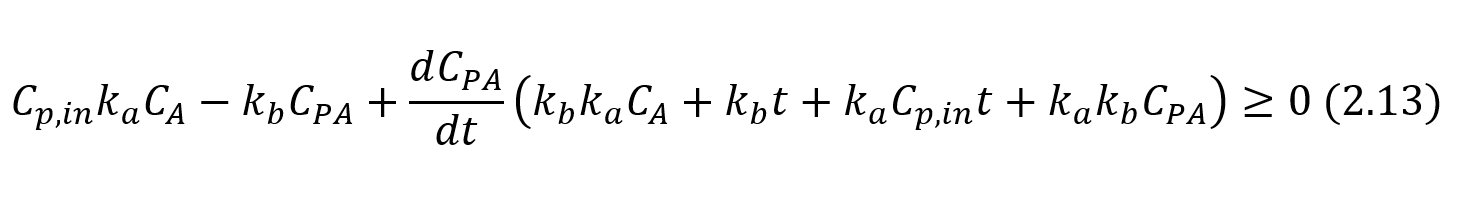We therefore have proven that:Two conclusions can be reached by analyzing inequation 2.14:

Conclusion a): the higher the flow rate v, the less efficient the protein can bind to the antibody, and the more unbinding protein will present in outflow.

Conclusion b): the wider the binding region is (the larger x is), the more efficient the protein can bind to the antibody.